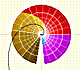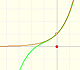The complex Exponential Function is the complex function that we can define as a power series and that extends the real Exponential function to complex valuesThe Exponential Function is periodic with period.

This series converges everywhere in the complex plane. Then the value of the function can be approximated by a partial sum (polynomial), and by choosing a sufficiently large degree we can make the approximation as accurate as we wish.

We can see it if we look at the Remainder (the difference between the function and the polynomial).

For example, this is one polynomial approximation of degree 6:And this is a representation of the remainder. The approximation is good but only near the center:We can see how the approximation improves when the polynomial is of degree 40:This is the remainder:The behavior of the power series of the complex exponential function is very good because the series converges everywhere. We can see a similar behavior studying the complex cosine function.

REFERENCES

Tristan Needham - Visual Complex Analysis. (pag. 80) - Oxford University Press